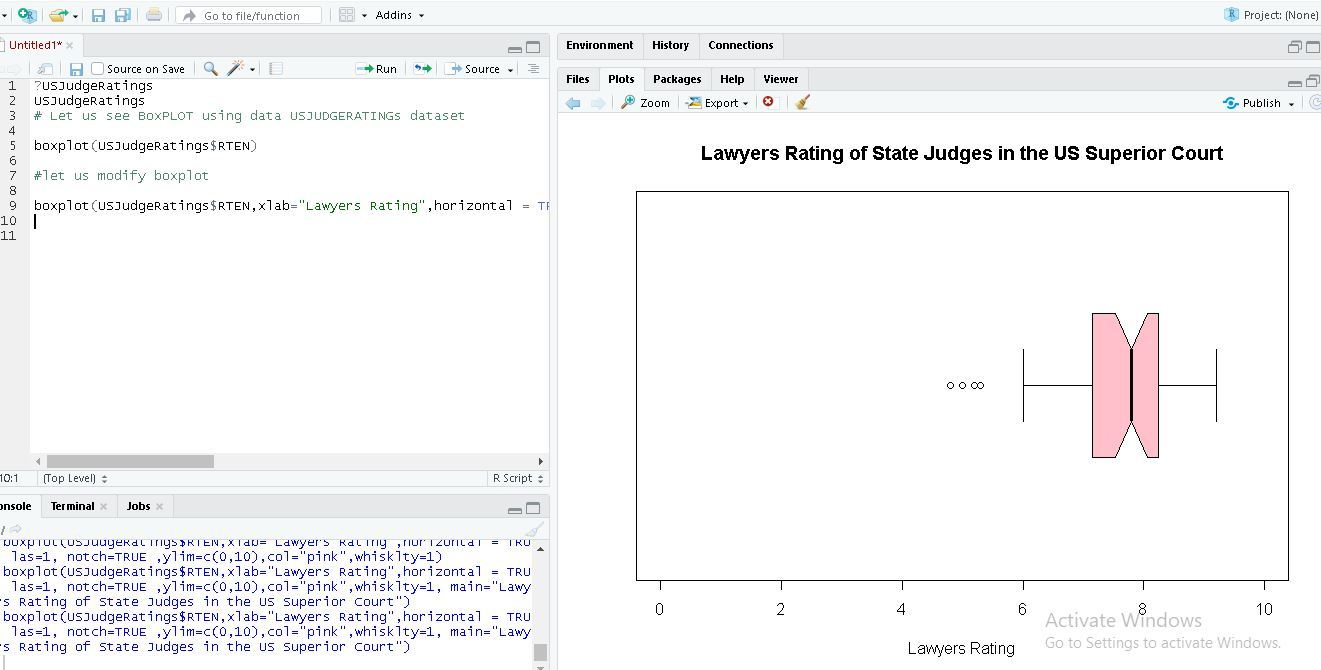Open In App
Related Articles
• Write an Interview Experience
• R Language Tutorial

# R – Statistics

Statistics is a form of mathematical analysis that concerns the collection, organization, analysis, interpretation, and presentation of data. The statistical analysis helps to make the best use of the vast data available and improves the efficiency of solutions.

## R – Statistics

R is a programming language and is used for environment statistical computing and graphics. The following is an introduction to basic statistical concepts like normal distribution (bell curve), central tendency (the mean, median, and mode), variability (25%, 50%, 75% quartiles), variance, standard deviation, modality, skewness.

## Data Concepts

Data can be formed in different structures and different formats, before starting the concepts of statistic we need to know the data formats.

These are some formats:

## Plotting graphs in Statistics in R Programming Language

Following is a list of functions that are required to plot graphs for the representation of Statistical data:

• plot() Function: This function is used to Draw a scatter plot with axes and titles.

Syntax:

plot(x, y = NULL, ylim = NULL, xlim = NULL, type = “b”….)

• data() function: This function is used to load specified data sets.

Syntax:

data(list = character(), lib.loc = NULL, package = NULL…..)

• table() Function: The table function is used to build a contingency table of the counts at each combination of factor levels.
`table(x, row.names = NULL, ...)`
• barplot() Function: It creates a bar plot with vertical/horizontal bars.

Syntax:

barplot(height, width = 1, names.arg = NULL, space = NULL…)

• pie() Function: This function is used to create a pie chart.

Syntax:

pie(x, labels = names(x), radius = 0.6, edges = 100, clockwise = TRUE …)

• hist() Function: The function hist() creates a histogram of the given data values.

Syntax:

hist(x, breaks = “Sturges”, probability = !freq, freq = NULL,…)

Note: You can find the information about each function using the “?” symbol before the beginning of each function.

R built-in datasets are very useful to start with and develop skills, So we will be using a few Built-in datasets. Let’s start by creating a simple bar chart by using chickwts dataset and learn how to use datasets and few functions of RStudio.

## Bar charts

A Bar chart represents categorical data with rectangular bars where the bars can be plotted vertically or horizontally.

## R

 `# ? is used before a function``# to get help on that function``?plot       ``?chickwts   ``data``(chickwts) ``#loading data into workspace``plot``(chickwts\$feed) ``# plot feed from chickwts`

In the above code ‘?’ in front of a particular function means that it gives information about that function with its syntax. In R ‘#’ is used for commenting single line and there is no multiline comment in R. Here we are using chickwts as the dataset and feed is the attribute in the dataset.

Output: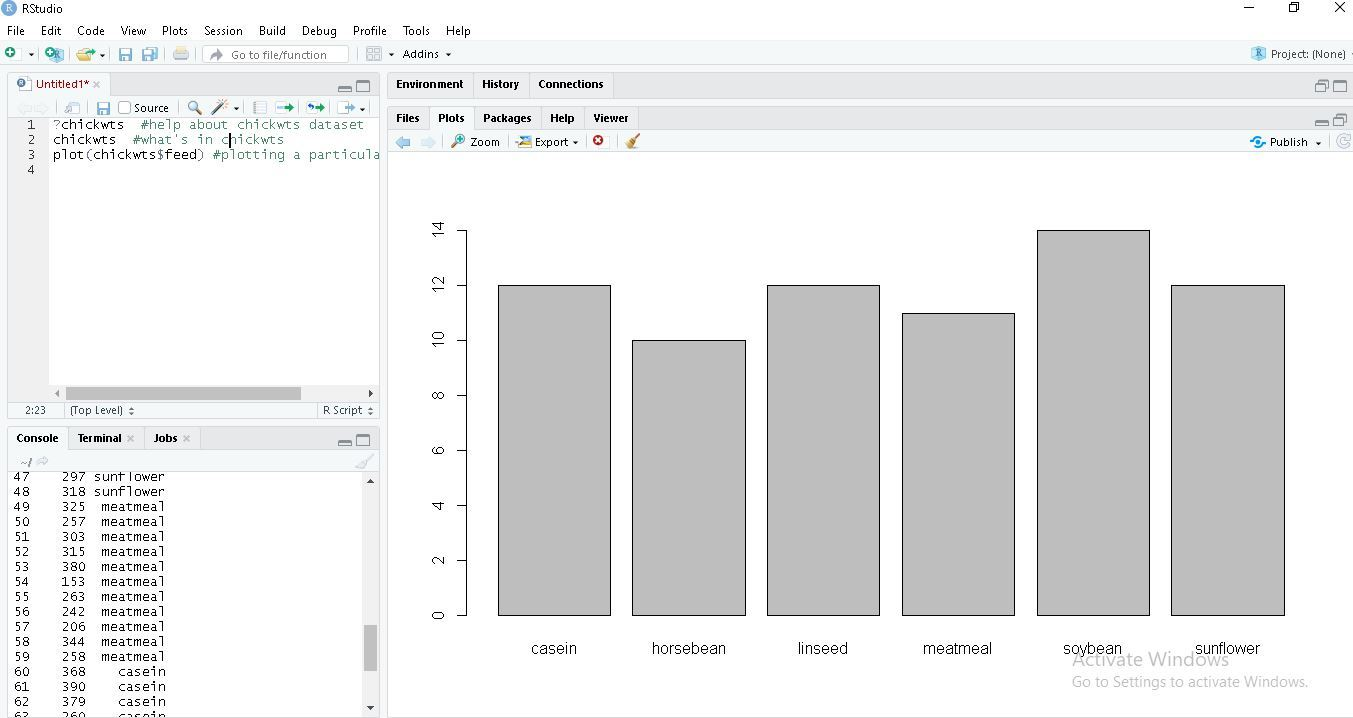## R

 `feeds=``table``(chickwts\$feed)` `# plots graph in decreasing order``barplot``(feeds[``order``(feeds, decreasing=``TRUE``)])`

Output: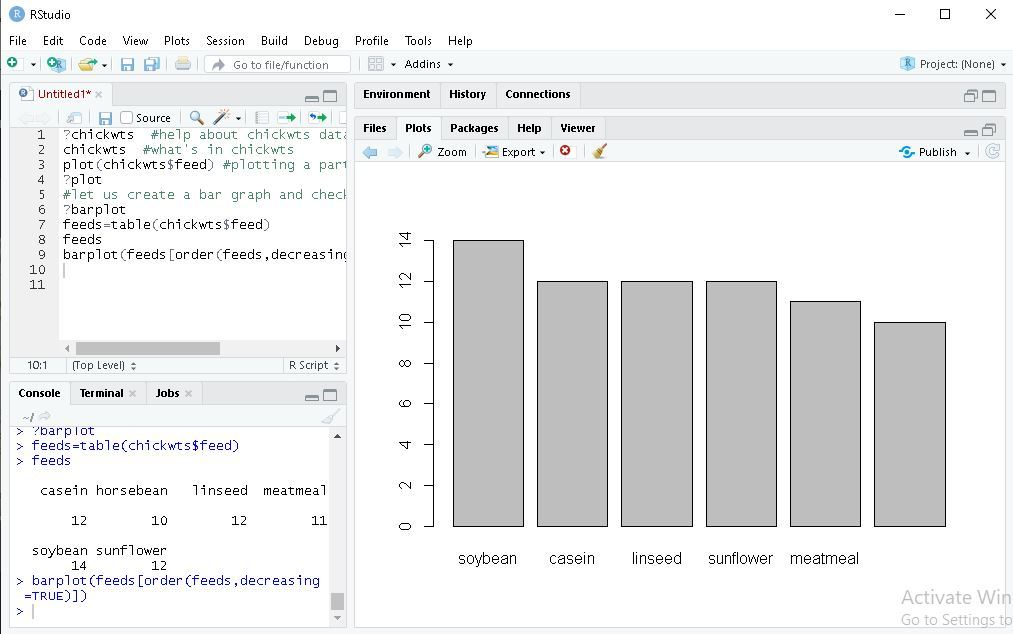## R

 `feeds = ``table``(chickwts\$feed)` `# outside margins bottom, left, top, right.``par``(oma=``c``(1, 1, 1, 1))                           ``par``(mar=``c``(4, 5, 2, 1))                           ` `# las is used orientation of axis labels   ``barplot``(feeds[``order``(feeds, decreasing=``TRUE``)]``    ` `# horiz is used for bars to be shown as horizontal.``barplot``(feeds[``order``(feeds)], horiz=``TRUE``,` `# col is used for colouring bars.   ``# xlab is used to label x-axis.``xlab=``"Number of chicks"``, las=1 col=``"yellow"``)   `

Output:## Pie charts

A pie chart is a circular statistical graph that is divided into slices to show the different sizes of the data.

## R

 `data``(``"chickwts"``)` `# main is used to create``# an heading for the chart``d = ``table``(chickwts\$feed)           ` `pie``(d[``order``(d, decreasing=``TRUE``)],``    ``clockwise=``TRUE``,``    ``main=``"Pie Chart of feeds from chichwits"``, )`

Output: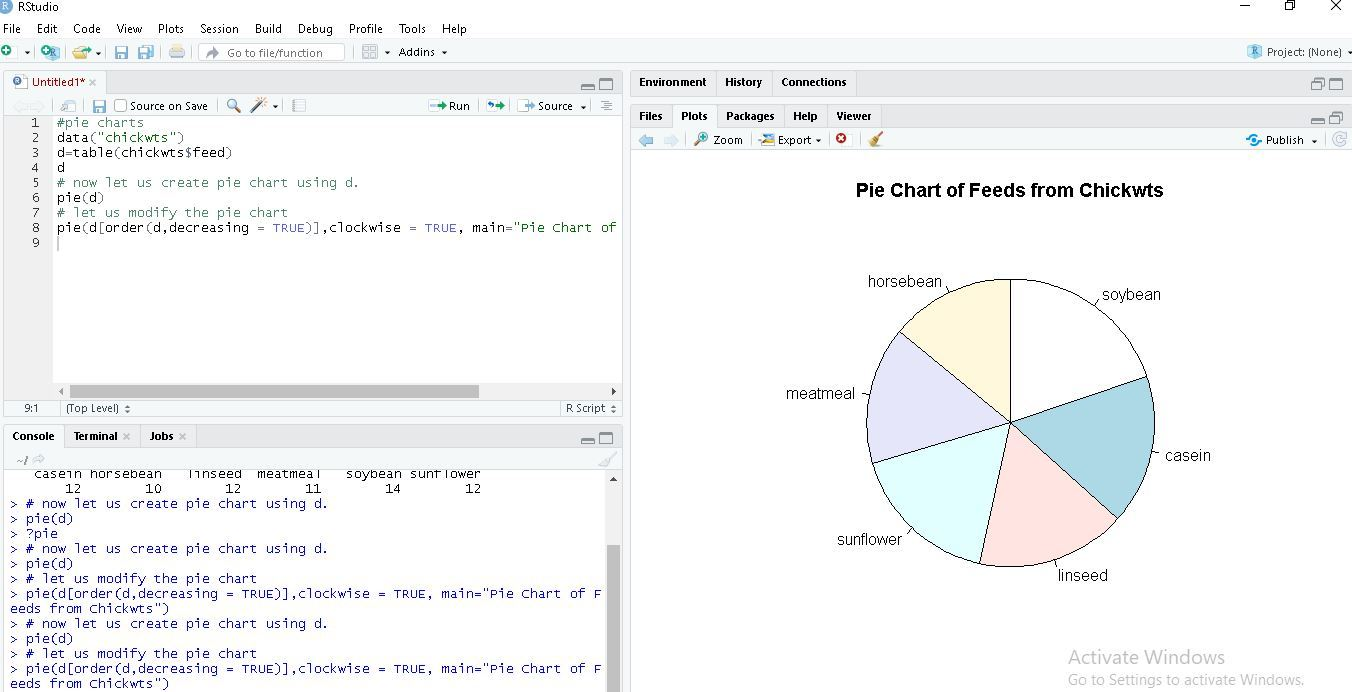## Histograms

Histograms are the representation of the distribution of data(numerical or categorical). It is similar to a bar chart but it groups data in terms of ranges.

## R

 `# break is used for number of bins.``data``(lynx)` `# lynx is a built-in dataset.``lynx       ` `# hist function is used to plot histogram.``hist``(lynx)``hist``(lynx, ``break``=7, col=``"green"``,``    ``main=``"Histogram of Annual Canadian Lynx Trappings"``)`

Output :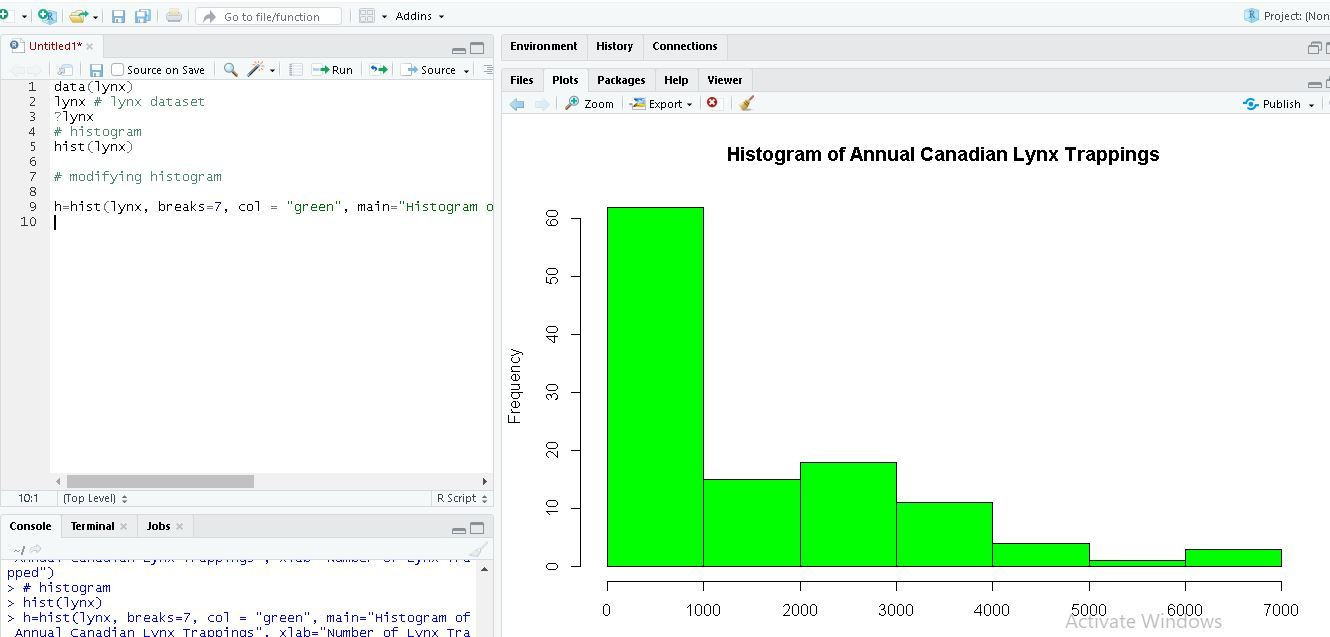## R

 `data``(lynx)` `# if freq=FALSE this will draw normal distribution``lynx               ``hist``(lynx)``hist``(lynx, ``break``=7, col=``"green"``,``    ``freq=``FALSE` `main=``"Histogram of Annual Canadian Lynx Trappings"``)` `curve``(``dnorm``(x, mean=``mean``(lynx),``            ``sd=``sd``(lynx)), col=``"red"``,``            ``lwd=2, add=``TRUE``)`

Output: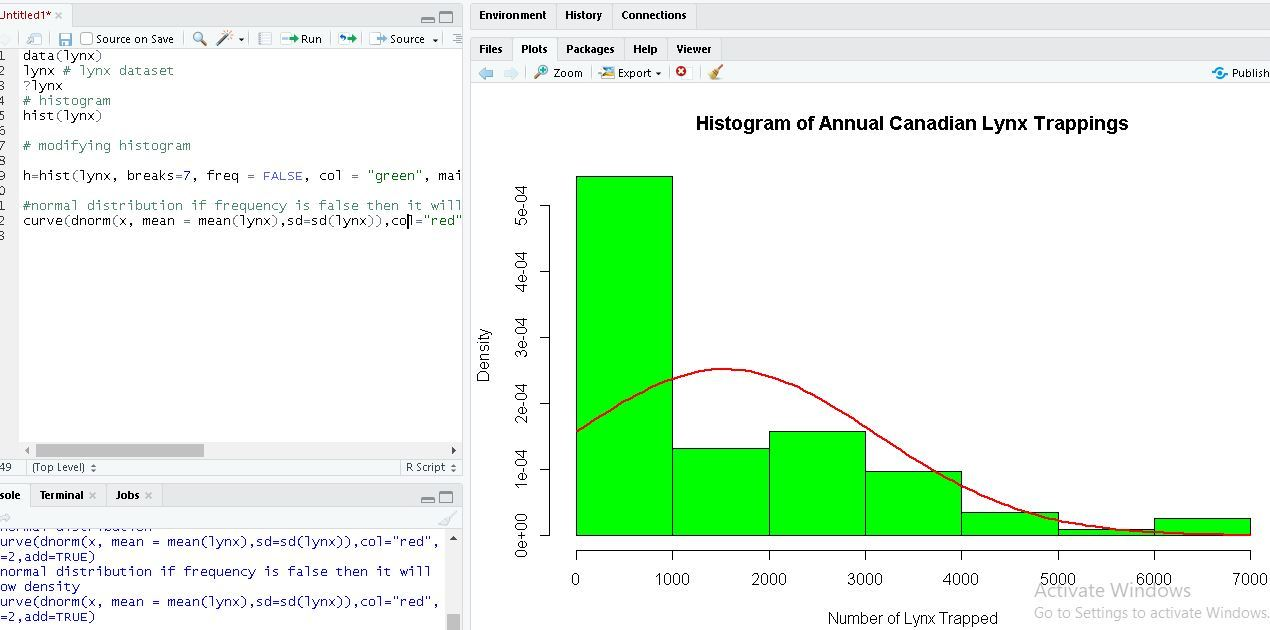## Box Plots

Box Plot is a function for graphically depicting groups of numerical data using quartiles. It represents the distribution of data and understanding mean, median, and variance.

## R

 `# USJudgeRatings is Built-in Dataset.``?USJudgeRatings                       ` `# ylim is used to specify the range.``boxplot``(USJudgeRatings\$RTEN, horizontal=``TRUE``,``        ``xlab=``"Lawyers Rating"``, notch=``TRUE``,``        ``ylim=``c``(0, 10), col=``"pink"``)`

USJudgeRating is a Build-in dataset with 6 attributes and RTEN is one of the attribute among it which is rating between 0 to 10 inclusive. We used it to for plotting a boxplot with different attributes of boxplot function.

Output: